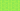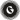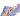PricingResources

# How to Calculate a Regression LineWritten by

A Least Squares Regression Line is frequently used in statistics to take a closer look at the relationship between data points. It’s also useful in business analysis, but what is a regression line exactly and how is it calculated? We’ll take a closer look at the formula and its applications below.

## What is a regression line?

A regression line displays the connection between scattered data points in any set. It shows the relation between the dependent y variable and independent x variables when there is a linear pattern. By calculating a regression line, analysts can then forecast the future behaviour of the dependent variable using different inputs.

## What is a regression line used for?

You’ll often see regression lines used in finance, whether it’s forecasting commodity prices or valuing securities. Businesses can also use regression lines to look at the relationships between different variables in accounting, such as sales, expenses, and inventory. A linear regression lets you use one variable to predict another variable’s value.

Regression line formula

The regression line formula used in statistics is the same used in algebra:

where:

• x = horizontal axis

• y = vertical axis

• m = the slope of the line (how steep it is)

• b = the y-intercept (where the line crosses the Y axis)

## How to calculate the regression line

One factor to keep in mind is that in statistics the points won’t lie perfectly straight on the line. Instead, you’re plotting a series of data points in a scatterplot formation and drawing the regression line with greatest possible accuracy between them. Although you can approximate this line by eye, using the regression line formula provides a higher degree of accuracy.

Calculating the least squares regression line offers a way to create a line that fits your data points with minimal variance. The least squares regression line minimises the vertical distance from each data point to the regression line, reducing error.

So, how do you put this formula into practice? First, you’ll need to calculate the values for m (slope) and b (y-intercept) so that you can plug them into the equation:

You’ll also need to calculate the following values before you can calculate a regression line:

• Mean of the x values

• Mean of the y values

• Standard deviation of x values

• Standard deviation of y values

• Correlation between x and y

Start by working out the slope, which represents the change in y over the change in x. For example, if the slope was 5/3, this means that the x-value would increase by 3 units and the y-value would increase by 5 units.

To calculate slope for a regression line, you’ll need to divide the standard deviation of y values by the standard deviation of x values and then multiply this by the correlation between x and y. The slope can be negative, which would show a line going downhill rather than upwards.

To find the y-intercept, you must then multiply the slope by the mean of the x values and then subtract this result from the mean of the y values.

With these results in hand, you can then plug them into the regression line formula and plot your results.

## Performing a regression analysis

Whether you use a regression line calculator or figure out the equation by hand, this is useful in performing a regression analysis for your business. Regression analysis looks at whether variables are related or dependent on one another. For example, you might wish to figure out to what degree your business sales are dependent on advertising costs. Create a hypothesis that you wish to test, gathering data points to analyse. In the example above, this might include advertising expenses and sales revenue pulled from financial statements.

You can then chart your data using a regression line calculator or spreadsheet app to show the correlation between these sets of variables. If you’re able to chart a straight regression line, this proves there is a positive correlation between the variables.

As you can see, learning to calculate a simple linear regression is a handy tool for analysis in business. You can put it to work to predict future revenue and expenses, looking for patterns and correlation in data points.

#### We can help

GoCardless helps you automate payment collection, cutting down on the amount of admin your team needs to deal with when chasing invoices. Find out how GoCardless can help you with ad hoc payments or recurring payments.Contact sales

Sales

Support